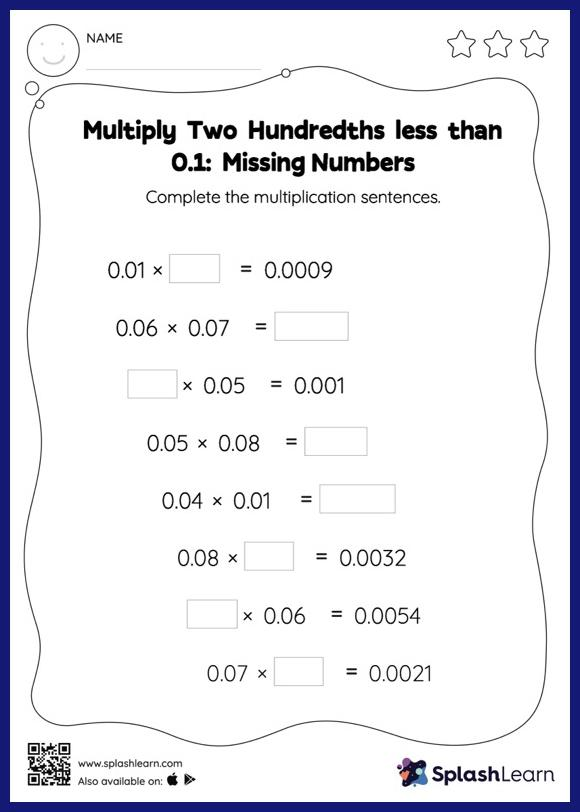# Multiply Two Hundredths less than 0.1: Missing Numbers Worksheet

Home > Multiply Two Hundredths less than 0.1: Missing NumbersWhen students multiply two decimals, they do so without considering the decimal point at first. They find the product and add the decimal point at the appropriate place in it. This approach helps them in finding the missing number in decimal multiplication as with multiply two hundredths less than 0.1 Worksheet. This worksheet is about practicing with the horizontal format in which numbers are written side by side. To develop flexibility with numbers and operations, students need to have enough practice in this format and not just rely on the vertical/column method.